安卓手机扫描二维码安装App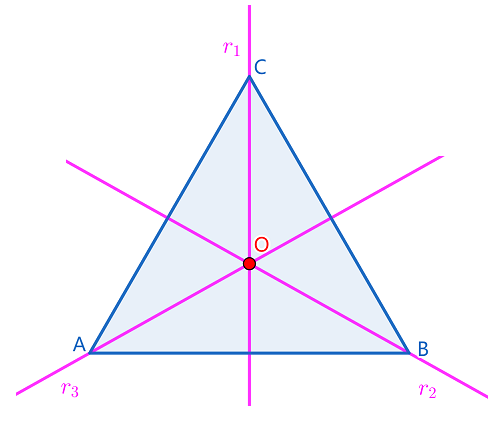(1)恒等变换，即不做任何操作，记作 ﻿$I$﻿ ;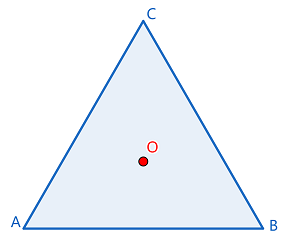(2)关于对称轴 ﻿$r_1$﻿ 所在直线的反射，记作 ﻿$r_1$﻿ ;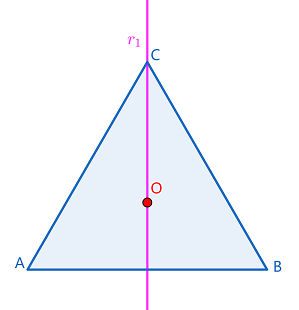(3)关于对称轴 ﻿$r_2$﻿ 所在直线的反射，记作 ﻿$r_2$﻿ ;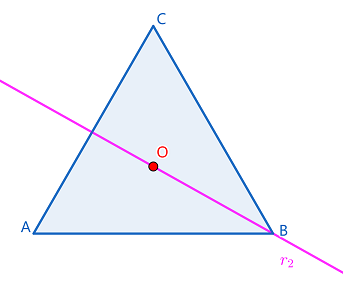(4)关于对称轴 ﻿$r_3$﻿ 所在直线的反射，记作 ﻿$r_3$﻿ ;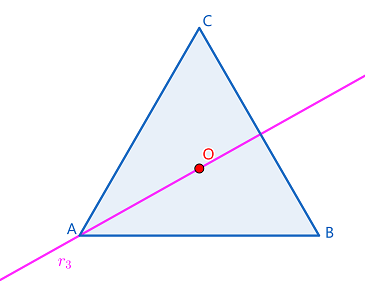(5)以点O为中心作 ﻿$120\degree$﻿ 旋转，记作 ﻿$\rho_1$﻿ ;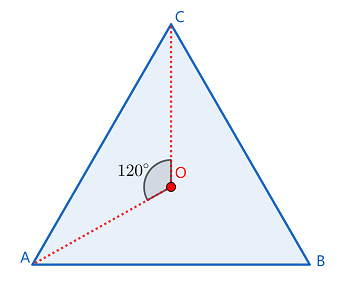(6)以点O为中心作 ﻿$240\degree$﻿ 旋转，记作 ﻿$\rho_2$﻿ .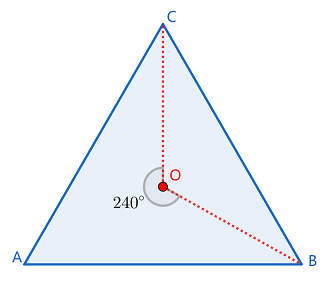﻿$D_3=$﻿ { ﻿$I,r_1,r_2,$﻿﻿$r_3,\rho_1,\rho_2$﻿ }

﻿$\rho_1 \cdot r_2=r_3$﻿

﻿$r_2 \cdot r_2 \cdot r_3$﻿

﻿$= r_2 \cdot \rho_1$﻿

﻿$= r_3$﻿

﻿$\rho_1 \cdot r_1$﻿ 是否等于 ﻿$r_1 \cdot \rho_1$﻿苹果手机扫描二维码安装App